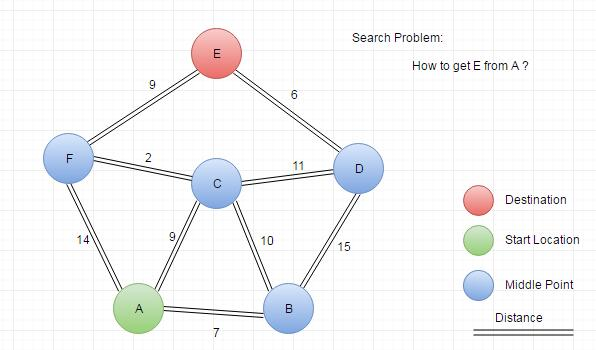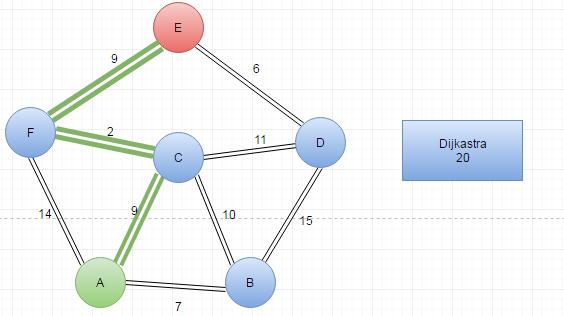Searching in a graph is the one of the most popular topic in CS.

In this article, I would like to make a summary about what algorithms for searching in graphes.

### 1. Presentation of Graph

What is better, adjacency lists or adjacency matrices for graph problem ?

It depends on the problem.

An adjacency matrix uses `O(n*n)` memory. It has fast lookups to check for presence or absence of a specific edge, but slow to iterate over all edges.

Adjacency lists use memory in proportion to the number edges, which might save a lot of memory if the adjacency matrix is sparse. It is fast to iterate over all edges, but finding the presence or absence specific edge is slightly slower than with the matrix.

In this article, I would like to use adjacency matrix to represent the graph in our problems. I want to express the essential idea in algorithms but not the programming language grammer. So all implementation of algorithm will be written in Python.

We will try to use different ways to solve the demo problem. Here is the graph which we will use in this article.Breadth-first search (BFS) is an algorithm for traversing or searching tree or graph data structures. It starts at the tree root (or some arbitrary node of a graph, sometimes referred to as a ‘search key’) and explores the neighbor nodes first, before moving to the next level neighbors.

Depth-first search (DFS) is an algorithm for traversing or searching tree or graph data structures. One starts at the root (selecting some arbitrary node as the root in the case of a graph) and explores as far as possible along each branch before backtracking.### Shortest Path Search (Dijkastra)Extention:

I will recommend you to finish the lab2 in 6.034

https://github.com/jasonleaster/MIT_6.034_2015/tree/master/lab2

This article does not finished and will be update these days :)

You can get my implementation on github

Photo by Annabella in ChongQin, China